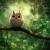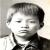## c语言，计算等式

Apria 发布于 2017/02/19 18:02

100

`123+45-67+8-9=100`

1``````#include <stdio.h>

char ans;
int num[] = {123,45,67,8,9};
int n;

int calc() {
int res = num;
for(int i = 0; i < 4; ++i) {
if(ans[i] == '+') res += num[1+i];
else res -= num[1+i];
}
return res;
}

void print_ans() {
printf("123%c45%c67%c8%c9=%d\n", ans, ans, ans, ans, n);
}

int solve(int depth) {
if(depth == 4) {
if(calc() == n) return 1;
else return 0;
}

ans[depth] = '+';
if(solve(depth+1)) return 1;
ans[depth] = '-';
if(solve(depth+1)) return 1;
return 0;
}

int main() {
while(scanf("%d", &n) == 1) {
if(solve(0)) print_ans();
else printf("impossible\n");
}
return 0;
}``````

0